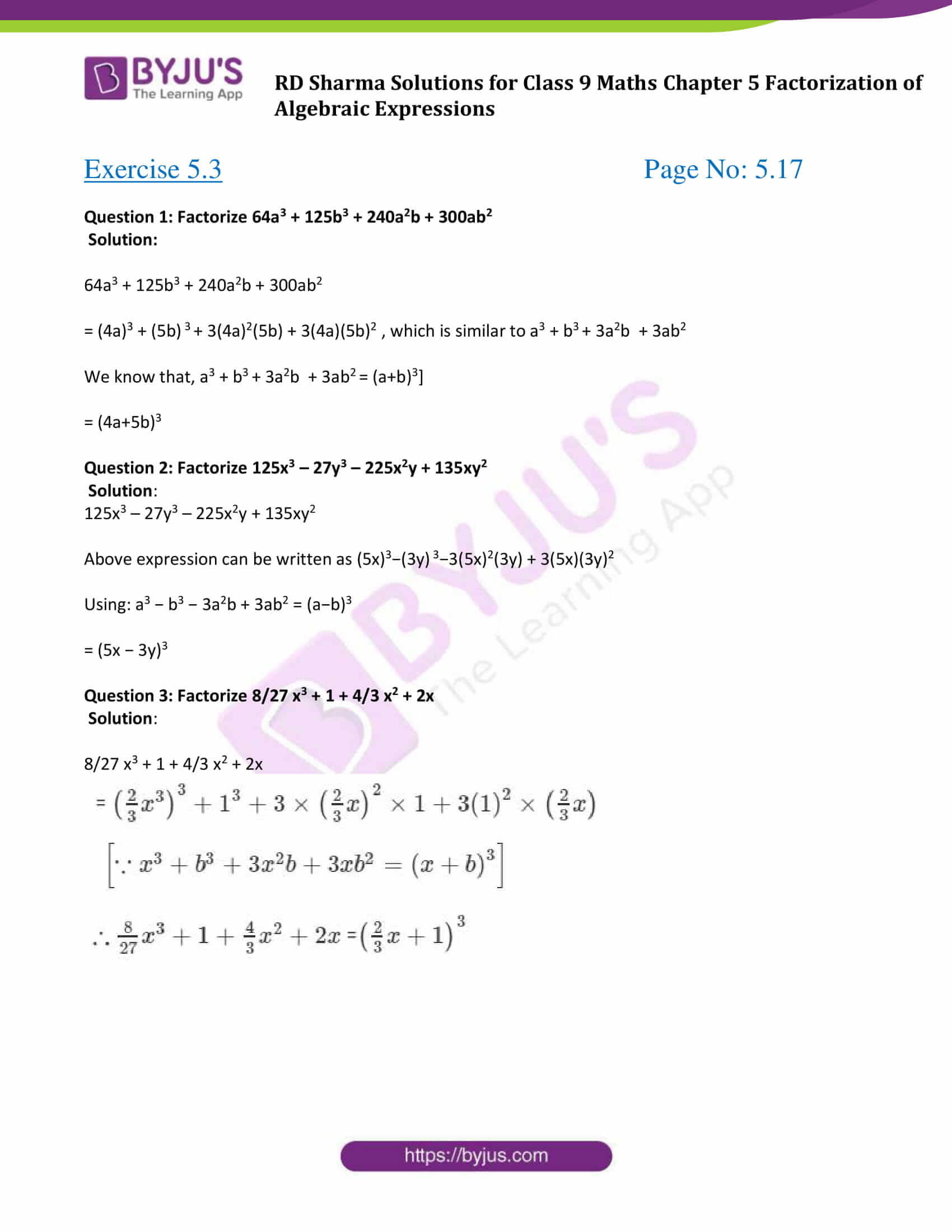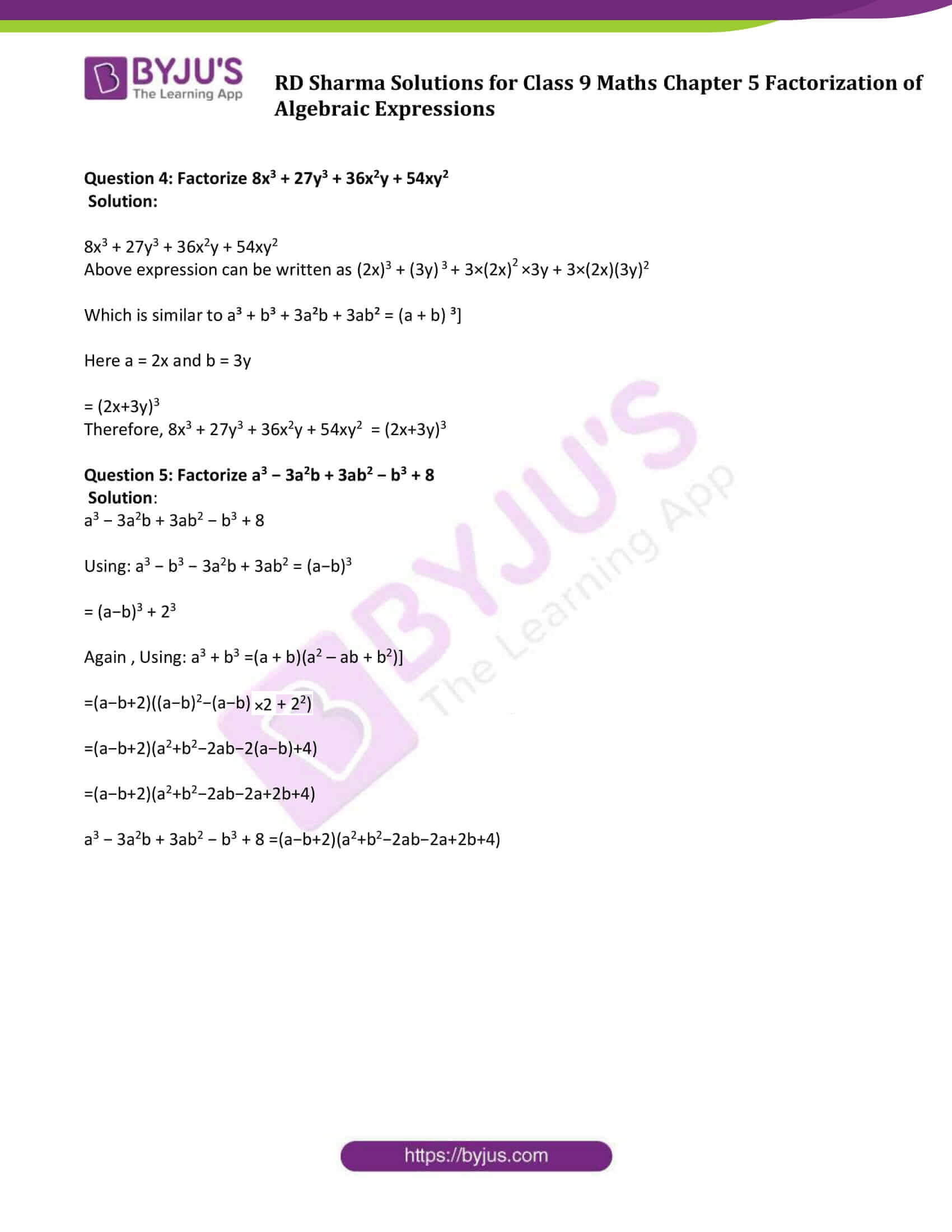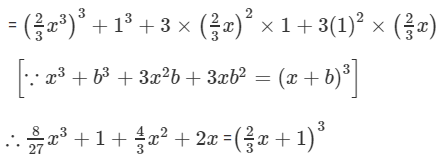# RD Sharma Solutions for Class 9 Maths Chapter 5 Factorization of Algebraic Expressions Exercise 5.3

RD Sharma Class 9 Mathematics Chapter 5 Exercise 5.3 Factorization of Algebraic Expressions is provided here. These exercise solutions are helpful to practice on factorization by using the formulae for the cube of a binomial. Students can enhance their knowledge quotient using the RD Sharma Solutions Class 9 chapter 5 and also get a clear overview of the topics. In this exercise, students will study the factorisation of algebraic expressions which are expressible in the form (x + y)3 or (x – y)3.

## Download PDF of RD Sharma Solutions for Class 9 Maths Chapter 5 Factorization of Algebraic Expressions Exercise 5.3### Access Answers to Maths RD Sharma Solutions for Class 9 Chapter 5 Factorization of Algebraic Expressions Exercise 5.3 Page number 5.17

#### Exercise 5.3 Page No: 5.17

Question 1: Factorize 64a3 + 125b3 + 240a2b + 300ab2

Solution:

64a3 + 125b3 + 240a2b + 300ab2

= (4a)3 + (5b) 3 + 3(4a)2(5b) + 3(4a)(5b)2 , which is similar to a3 + b3 + 3a2b + 3ab2

We know that, a3 + b3 + 3a2b + 3ab2 = (a+b)3]

= (4a+5b)3

Question 2: Factorize 125x3 – 27y3 – 225x2y + 135xy2

Solution:

125x3 – 27y3 – 225x2y + 135xy2

Above expression can be written as (5x)3−(3y) 3−3(5x)2(3y) + 3(5x)(3y)2

Using: a3 − b3 − 3a2b + 3ab2 = (a−b)3

= (5x − 3y)3

Question 3: Factorize 8/27 x3 + 1 + 4/3 x2 + 2x

Solution:

8/27 x3 + 1 + 4/3 x2 + 2xQuestion 4: Factorize 8x3 + 27y3 + 36x2y + 54xy2

Solution:

8x3 + 27y3 + 36x2y + 54xy2

Above expression can be written as (2x)3 + (3y) 3 + 3×(2x)2×3y + 3×(2x)(3y)2

Which is similar to a³ + b³ + 3a²b + 3ab² = (a + b) ³]

Here a = 2x and b = 3y

= (2x+3y)3

Therefore, 8x3 + 27y3 + 36x2y + 54xy2 = (2x+3y)3

Question 5: Factorize a3 − 3a2b + 3ab2 − b3 + 8

Solution:

a3 − 3a2b + 3ab2 − b3 + 8

Using: a3 − b3 − 3a2b + 3ab2 = (a−b)3

= (a−b)3 + 23

Again , Using: a3 + b3 =(a + b)(a2 – ab + b2)]

=(a−b+2)((a−b)2−(a−b)×2 + 22)

=(a−b+2)(a2+b2−2ab−2(a−b)+4)

=(a−b+2)(a2+b2−2ab−2a+2b+4)

a3 − 3a2b + 3ab2 − b3 + 8 =(a−b+2)(a2+b2−2ab−2a+2b+4)

## RD Sharma Solutions for Class 9 Maths Chapter 5 Factorization of Algebraic Expressions Exercise 5.3

RD Sharma Solutions Class 9 Maths Chapter 5 Factorization of Algebraic Expressions Exercise 5.3 is based on the following topic:

• Factorisation by using the formulae for the cube of a binomial

(x + y)3 = x3 + y3 + 3xy(x + y)

(x – y)3 = x3 – y3 – 3xy(x – y)